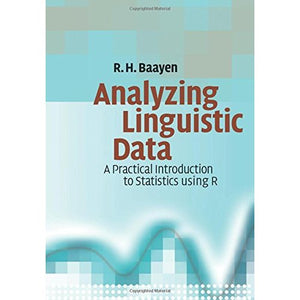># Analyzing Linguistic Data: A Practical Introduction to Statistics using R

• £6.19
• Save £30

R. H. Baayen
Cambridge University Press, 3/6/2008
EAN 9780521709187, ISBN10: 0521709180

Paperback, 368 pages, 24.4 x 17 x 2.1 cm
Language: English

Statistical analysis is a useful skill for linguists and psycholinguists, allowing them to understand the quantitative structure of their data. This textbook provides a straightforward introduction to the statistical analysis of language. Designed for linguists with a non-mathematical background, it clearly introduces the basic principles and methods of statistical analysis, using 'R', the leading computational statistics programme. The reader is guided step-by-step through a range of real data sets, allowing them to analyse acoustic data, construct grammatical trees for a variety of languages, quantify register variation in corpus linguistics, and measure experimental data using state-of-the-art models. The visualization of data plays a key role, both in the initial stages of data exploration and later on when the reader is encouraged to criticize various models. Containing over 40 exercises with model answers, this book will be welcomed by all linguists wishing to learn more about working with and presenting quantitative data.

1. An introduction to 'R'
2. Graphic data exploration
3. Probability distributions
4. Basic statistical methods
5. Clustering and classification
6. Regression modeling
7. Mixed models
Appendix A. Solutions to the exercises
Appendix B. Overview of 'R' functions.